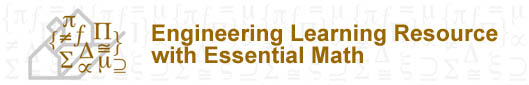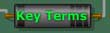Learning Resource Topics Overview 1 Charge & Coulomb’s Law 2 Electric Current 3 Voltage 4 Resistance 5 Ohm’s Law 6 Power & Energy 7 Elements of Electric Circuits 8 Kirchhoff’s Law 9 Series Circuits 10 Parallel Circuits 11 Analysis of Combination Circuits 12 Capacitance 13 Inductance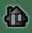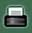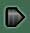# REVIEW QUESTIONS

1. What is matter, and in what three states is it found?
2. What is an element?
3. What is a coulomb?
4. What is Coulomb’s law? State its properties.
5. What determines whether a substance is a conductor or an insulator?
6. What is an ampere?
7. Define voltage.
8. Define Ohm.
9. What is the relationship of current to voltage in a circuit?
10. Are all the electrons flowing in a circuit provided by the battery?
11. Why must there be no gaps in an electric circuit for it to carry current?
12. Differentiate between a series circuit and a parallel circuit.
13. If two lamps are connected in series to a 9-V battery, how many volts are impressed across each lamp?
14. If one of two lamps blows out when connected in series, what happens to the current in the other two?
15. If two lamps are connected in parallel to a 9-V battery, how many volts are impressed across each lamp?
16. If one of two lamps blows out when connected in parallel, what happens to the current in the other one?
17. In which case will there be more current in each of two lamps—if they are connected to the same battery in series or in parallel?
18. In which case will there be more voltage across each lamp
19. What happens to the total circuit resistance when more devices are added to a series circuit? To a parallel circuit?
20. What is the equivalent resistance of a pair of 4-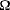resistors in series? In parallel?
21. Why does the total circuit resistance decrease when more devices are added to a parallel circuit?
22. What does it mean when you say that lines in a home are overloaded?
23. What is the function of a fuse or circuit breaker in a circuit?
24. What is meant by a short circuit?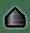Content and Pedagogy© 2004, University of Ottawa, Faculty of Engineering and Faculty of Education
Design and Production © 2004, University of Ottawa, Centre for e-Learning